# Expectation propagation

## Notation

•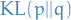denotes the Kullback-Leibler divergence, to be minimized wrt.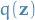•is the approximate distribution, which is a member of the exponential family, i.e. can be written: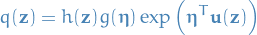•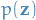is a fixed distribution
•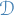denotes the data
•denotes the parameters / hidden variables

## Exponential family

The exponential family of distributions over, given parameters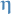, is defined to be the set of distribtions of the form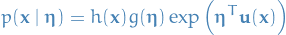where:

•may be discrete or continuous
•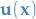is just some function of### MLE

If we wanted to estimatein a density of the exponential family, we would simply take the derivative wrtof the likelihood of the distribution and set to zero: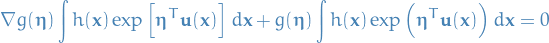Rearranging, we get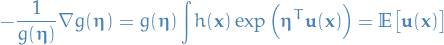which is just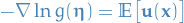The covariance ofcan be expressed in terms of the second derivatives of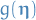, and similarily for higher order moments.

Thus, if we had set of i.i.d. data denoted by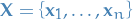, for which the likelihood function is given by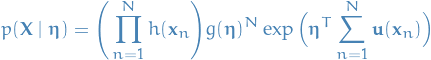which is minimized wrt.when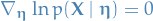giving us the MLE estimator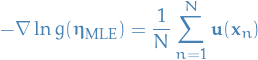i.e. the MLE estimator depends on the data only through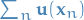, henceis a sufficient statistic.## Minimizing KL-divergence between two exponential distributions

The KL divergence then becomes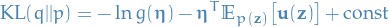where,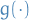and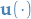define the approximation distribution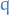.

We're not making any assumptions regarding the underlying distribution of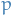.

Which is minimized wrt.by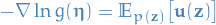From MLE estimator for exponential we then have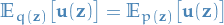hence, the optimum solution corresponds to matching the expected sufficient statistics!

## Expectation propagation

• Joint probability of data and parameters given by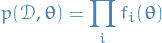• Want to evaluate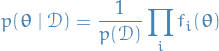and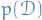for model comparison: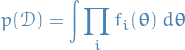• Expectation propagation is based on the approximation to the posterior distribution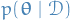by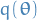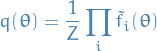where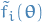is an approximation to the factor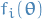in the true posterior

• To be tractable, need to constrain the approximators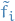=> assume to be exponential
• Want to determineby minimizing KL divergence between true and approx. posterior: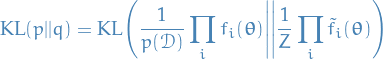One approach of doing this would be to minimize KL divergence between the pairsandof factors.

It turns out that this no good; even though each of the factors are approximated individually, the product could still give a poor approximation.

(Also, if our assumptions are not true, i.e. true posterior is actually not of the exponential family, then clearly this approach would not be a good one.)

Expectation propagation optimizes each factorin turn, given the "context" of all remaining factors.

Suppose we wish to update our estimate for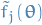, then we would like the following (assuming data was drawn from some exponential distribution):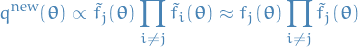i.e. want to optimize for the j-th factor conditioned on our estimate for all the other factors. (Expectation maximization, anyone?)

This problem we can pose as minimizing the following KL divergence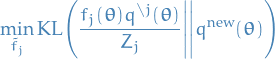where:

• new approximate distribution: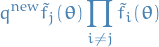• the unnormalized distribution: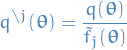• normalization with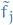replaced by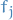: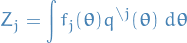Which we already know comes down to having:and we wish to approximate the posterior distributionby a distribution of the formand the model evidence.

1. Initialize all of the approximating factors2. Initialize the posterior approximation by setting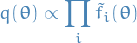3. Until convergence:
1. Choose a factorto improve
2. Removefrom posterior by division:3. Let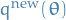be such that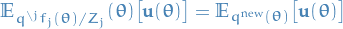i.e. matching the sufficient statistics (moments) ofwith those of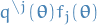, including evaluating the normalization constant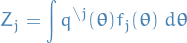4. Evaluate and store new factor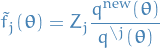4. Evaluate the approximation to the model evidence: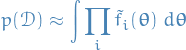### Notes

• No guarantee that iterations will converge
• Fixed-points guaranteed to exist when the approximations are in the exponential family
• So at least then it converges, but to what? Dunno
• Can also have multiple fixed-points
• However, if iteratiors do converge; resulting solution will be a stationary point of a particular energy function (although each iteration is not guaranteed to actually decrease this energy function)
• Does not make sense to apply EP to mixtures as the approximation tries to capture all of the modes of the posterior distribution

### Moment matching / Assumed density filtering (ADF): a special case of EP

• Initializes allto unity and performs a single pass through the approx. factors, updating each of them once
• Does not make use of batching
• Highly dependent on order of data points, as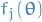are only updated once